We’d like to understand how you use our websites in order to improve them. Register your interest.

# On pointwise and uniform statistical convergence of order α for sequences of functions

## Abstract

In this paper, we introduce the concepts of pointwise and uniform statistical convergence of order α for sequences of real-valued functions. Furthermore, we give the concept of an α-statistically Cauchy sequence for sequences of real-valued functions and prove that it is equivalent to pointwise statistical convergence of order α for sequences of real-valued functions. Also, some relations between $S α (f)$-statistical convergence and strong $w p β (f)$-summability are given.

MSC:40A05, 40C05, 46A45.

## 1 Introduction

The idea of statistical convergence was given by Zygmund  in the first edition of his monograph published in Warsaw in 1935. The concept of statistical convergence was introduced by Steinhaus  and Fast  and later reintroduced by Schoenberg  independently. Over the years and under different names, statistical convergence has been discussed in the theory of Fourier analysis, ergodic theory, number theory, measure theory, trigonometric series, turnpike theory and Banach spaces. Later on it was further investigated from the sequence space point of view and linked with summability theory by Başar , Connor , Et et al. , Fridy , Güngör et al. , Işık [12, 13], Kolk , Mohiuddine et al. , Miller and Orhan , Mursaleen , Rath and Tripathy , Salat , Savaş  and many others. In recent years, generalizations of statistical convergence have appeared in the study of strong integral summability and the structure of ideals of bounded continuous functions on locally compact spaces. Statistical convergence and its generalizations are also connected with subsets of the Stone-Čech compactification of the natural numbers. Moreover, statistical convergence is closely related to the concept of convergence in probability.

The definitions of pointwise and uniform statistical convergence of sequences of real-valued functions were given by Gökhan et al. [25, 26] and independently by Duman and Orhan . In the present paper, we introduce and examine the concepts of pointwise and uniform statistical convergence of order α for sequences of real-valued functions. In Section 2 we give a brief overview of statistical convergence of order α and strong p-Cesàro summability. In Section 3 we give the concepts of pointwise and uniform statistical convergence of order α, and the concept α-statistically Cauchy sequence for sequences of real-valued functions and prove that it is equivalent to pointwise statistical convergence of order α for sequences of real-valued functions. We also establish some inclusion relations between $w p β (f)$ and $S α (f)$ and between $S α (f)$ and $S(f)$.

## 2 Definition and preliminaries

The definitions of statistical convergence and strong p-Cesàro convergence of a sequence of real numbers were introduced in the literature independently of one another and have followed different lines of development since their first appearance. It turns out, however, that the two definitions can be simply related to one another in general and are equivalent for bounded sequences. The idea of statistical convergence depends on the density of subsets of the set of natural numbers. The density of a subset E of is defined bywhere $χ E$ is the characteristic function of E. It is clear that any finite subset of has zero natural density and $δ( E c )=1−δ(E)$.

The α-density of a subset E of was defined by Çolak . Let α be a real number such that $0<α≤1$. The α-density of a subset E of is defined by

$δ α (E)= lim n 1 n α |{k≤n:k∈E}|provided the limit exists,$

where $|{k≤n:k∈E}|$ denotes the number of elements of E not exceeding n.

If $x=( x k )$ is a sequence such that $x k$ satisfies property $P(k)$ for almost all k except a set of α-density zero, then we say that $x k$ satisfies property $P(k)$ for ‘almost all k according to α’ and we abbreviate this by ‘a.a.k $(α)$’.

It is clear that any finite subset of has zero α density and $δ α ( E c )=1− δ α (E)$ does not hold for $0<α<1$ in general, the equality holds only if $α=1$. Note that the α-density of any set reduces to the natural density of the set in case $α=1$.

The order of statistical convergence of a sequence of numbers was given by Gadjiev and Orhan in , and after then statistical convergence of order α and strong p-Cesàro summability of order α were studied by Çolak .

The statistical convergence of order α is defined as follows. Let $0<α≤1$ be given. The sequence $( x k )$ is said to be statistically convergent of order α if there is a real number such that

$lim n → ∞ 1 n α | { k ≤ n : | x k − ℓ | ≥ ε } |=0,$

for every $ε>0$, in which case we say that x is statistically convergent of order α to . In this case, we write $S α −lim x k =ℓ$. The set of all statistically convergent sequences of order α will be denoted by $S α$. We write $S 0 α$ to denote the set of all statistically null sequences of order α. It is clear that $S 0 α ⊂ S α$ for each $0<α≤1$. The statistical convergence of order α is same with the statistical convergence for $α=1$.

A sequence $x=( x k )$ is said to be strongly Cesàro summable to a number if $lim n 1 n × ∑ k = 1 n | x k −ℓ|=0$. The set of strongly Cesàro summable sequences is denoted by $[C,1]$ and defined as

There is a natural relationship between statistical convergence and strong p-Cesàro summability.

## 3 Main result

In this section we give the main results of this article. We give relations between the statistical convergence of order α and the statistical convergence of order β for sequences of functions, the relations between the strong p-Cesàro summability of order α and the strong p-Cesàro summability of order β and the relations between the strong p-Cesàro summability of order α and the statistical convergence of order β for sequences of real-valued functions, where $α≤β$.

Definition 3.1 Let $0<α≤1$ be given. A sequence of functions ${ f k }$ is said to be pointwise statistically convergent of order α (or pointwise α-statistically convergent sequence) to the function f on a set A if, for every $ε>0$,

i.e., for every $x∈A$,

$| f k (x)−f(x)|<ε a.a.k (α).$
(1)

In this case, we write $S α −lim f k (x)=f(x)$ on A. $S α −lim f k (x)=f(x)$ means that for every $δ>0$ and $0<α≤1$, there is an integer N such that

for all $n>N$ ($=N(ε,δ,x)$) and for each $ε>0$. The set of all pointwise statistically convergent sequences of functions order α will be denoted by $S α (f)$. For $α=1$, we will write $S(f)$ instead of $S α (f)$ and in the special case $f=0$, we will write $S 0 α (f)$ instead of $S α (f)$.

The statistical convergence of order α for a sequence of functions is well defined for $0<α≤1$. But it is not well defined for $α>1$. For this, let ${ f k }$ be defined as follows:

$f k (x)={ 1 , k = 2 n , x k , k ≠ 2 n , n=1,2,3,…,x∈ [ 0 , 1 2 ] .$

Then both

and

for $α>1$, so that ${ f k }$ statistically converges of order α both to 1 and 0, i.e., $S α −lim f k (x)=1$ and $S α −lim f k (x)=0$, which is impossible.

Theorem 3.2 Let $0<α≤1$ and ${ f k }$, ${ g k }$ be sequences of real-valued functions defined in a set A.

1. (i)

If $S α −lim f k (x)=f(x)$ and $c∈R$, then $S α −limc f k (x)=cf(x)$.

2. (ii)

If $S α −lim f k (x)=f(x)$ and $S α −lim g k (x)=g(x)$, then $S α −lim( f k (x)+ g k (x))=f(x)+g(x)$.

Proof (i) The proof is clear in case $c=0$. Suppose that $c≠0$ and $S α −lim f k (x)=f(x)$, then there exists $ε>0$ such that

$| f k (x)−f(x)|< ε | c | a.a.k (α),$

and hence

$|c f k (x)−cf(x)|<ε a.a.k (α).$

This implies that $S α −limc f k (x)=cf(x)$.

The proof of (ii) follows from the following inequalities: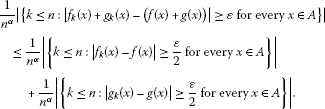It is easy to see that every convergent sequence of functions is statistically convergent of order α, that is, $c(f)⊂ S α (f)$ for each $0<α≤1$. But the converse of this does not hold. For example, the sequence ${ f k }$ defined by

$f k (x)={ 1 , k = n 3 , 2 k x 1 + k 2 x 2 , k ≠ n 3$

is statistically convergent of order α with $S α −lim f k (x)=0$ for $α> 1 3$, but it is not convergent. □

Definition 3.3 Let α be any real number such that $0<α≤1$ and let ${ f k }$ be a sequence of functions on a set A. The sequence ${ f k }$ is a statistically Cauchy sequence of order α (or α-statistically Cauchy sequence) provided that for every $ε>0$, there exists a number N ($=N(ε,x)$) such that

$| f k (x)− f N (x)|<ε a.a.k (α),$

i.e.,

Theorem 3.4 Let ${ f k }$ be a sequence of functions defined on a set A. The following statements are equivalent:

1. (i)

${ f k }$ is a pointwise α-statistically convergent sequence on A;

2. (ii)

${ f k }$ is a α-statistically Cauchy sequence on A;

3. (iii)

${ f k }$ is a sequence of functions for which there is a pointwise convergent sequence of order α, a sequence of functions ${ g k }$ such that $f k (x)= g k (x)$ a.a.k $(α)$ for every $x∈A$.

Proof (i) (ii) Suppose that $S α −lim f k (x)=f(x)$ on A and let $ε>0$. Then $| f k (x)−f(x)|< ε 2$ a.a.k $(α)$ and if N is chosen so that $| f N (x)−f(x)|< ε 2$, then we have

$| f k (x)− f N (x)|≤| f k (x)−f(x)|+| f N (x)−f(x)|< ε 2 + ε 2 a.a.k (α)$

for every $x∈A$. Hence ${ f k }$ is an α-statistically Cauchy sequence.

Next, assume (ii) is true and choose N so that the band $I=[ f N (x)−1, f N (x)+1]$ contains $f k (x)$ a.a.k $(α)$ for every $x∈A$. Also, apply (ii) to choose M so that $I ′ =[ f M (x)− 1 2 , f M (x)+ 1 2 ]$ contains $f k (x)$ a.a.k $(α)$ for every $x∈A$. We assert that

for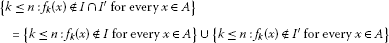so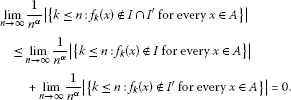Therefore, $I 1$ is a closed band of height less than or equal to 1 that contains $f k (x)$ a.a.k $(α)$ for every $x∈A$. Now we proceed by choosing $N(2)$ so that $I ″ =[ f N ( 2 ) (x)− 1 4 , f N ( 2 ) (x)+ 1 4 ]$ contains $f k (x)$ a.a.k $(α)$, and by the preceding argument, $I 2 = I 1 ∩ I ″$ contains $f k (x)$ a.a.k $(α)$ for every $x∈A$ and $I 2$ has height less than or equal to $1 2$. Continuing inductively, we construct a sequence ${ I m } m = 1 ∞$ of closed band such that for each m, $I m ⊇ I m + 1$, the height of $I m$ is not greater than $2 1 − m$and $f k (x)∈ I m$ a.a.k $(α)$ for every $x∈A$. Thus there exists a function $f(x)$, defined on A, such that ${f(x)}$ is equal to $⋂ m = 1 ∞ I m$. Using the fact that $f k (x)∈ I m$ a.a.k $(α)$ for every $x∈A$, we choose an increasing positive integer sequence ${ T m } m = 1 ∞$ such that

(2)

Now define a subsequence $( z k (x))$ of $( f k (x))$ consisting of all terms $f k (x)$ such that $k> T 1$ and if $T m then $f k (x)∉ I m$ for every $x∈A$. Next, define the sequence of functions $( g k (x))$ by

for every $x∈A$. Then $lim k → ∞ g k (x)=f(x)$ on A; for if $ε> 1 m >0$ and $k> T m$, then either $f k (x)$ is a term of $( z k (x))$ or $g k (x)= f k (x)∈ I m$ on A and $| g k (x)− f k (x)|≤$ height of $I m ≤ 2 1 − m$ for every $x∈A$. We also assert that $g k (x)= f k (x)$ a.a.k $(α)$ for every $x∈A$. To verify this, we observe that if $T m , then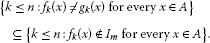So, by (2)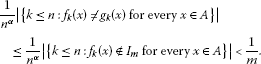Hence, the limit is 0 as $n→∞$ and $f k (x)= g k (x)$ a.a.k $(α)$ for every $x∈A$. Therefore, (ii) implies (iii).

Finally, assume that (iii) holds, say $f k (x)= g k (x)$ a.a.k $(α)$ for every $x∈A$ and $lim k → ∞ g k (x)=f(x)$ on A. Let $ε>0$. Then for each n,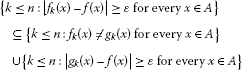since $lim k → ∞ g k (x)=f(x)$ on A, the latter set contains a fixed number of integers, say $l=l(ε,x)$. Therefore,because $f k (x)= g k (x)$ a.a.k $(α)$ for every $x∈A$. Hence $| f k (x)−f(x)|<ε$ a.a.k $(α)$ for every $x∈A$, so (i) holds and the proof is complete. □

Corollary 3.5 If ${ f k }$ is a sequence of functions such that $S α −lim f k (x)=f(x)$ on A, then ${ f k }$ has a subsequence ${ f k ( n ) (x)}$ such that $lim n → ∞ f k ( n ) (x)=f(x)$ on A.

Theorem 3.6 Let $0<α≤β≤1$. Then $S α (f)⊆ S β (f)$ and the inclusion is strict for some α and β such that $α<β$.

Proof If $0<α≤β≤1$, then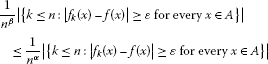for every $ε>0$ and this gives that $S α (f)⊆ S β (f)$. To show that the inclusion is strict, consider the sequence ${ f k }$ defined by

$f k (x)={ 1 , k = n 2 , k 2 x 1 + k 3 x 2 , k ≠ n 2 , n=1,2,3,…,x∈[0,1].$

Hence we can write for $1 2 <α≤1$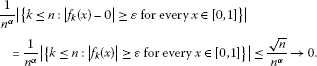Then $S β −lim f k (x)=0$, i.e., $x∈ S β (f)$ for $1 2 <β≤1$, but $x∉ S α (f)$ for $0<α≤ 1 2$. □

If we take $β=1$ in Theorem 3.6, then we obtain the following result.

Corollary 3.7 If a sequence of functions ${ f k }$ is statistically convergent of order α, to the function f for some $0<α≤1$, then it is statistically convergent to the function f.

Definition 3.8 Let α be any real number such that $0<α≤1$ and let p be a positive real number. A sequence of functions ${ f k }$ is said to be strongly p-Cesàro summable of order α if there is a function f such that

$lim n → ∞ 1 n α ∑ k = 1 n | f k (x)−f(x) | p =0.$

In this case, we write $w p α −lim f k (x)=f(x)$ on A. The strong p-Cesàro summability of order α reduces to the strong p-Cesàro summability for $α=1$. The set of all strongly p-Cesàro summable sequences of functions of order α will be denoted by $w p α (f)$. We write $w o , p α (f)$ in case $f(x)=0$.

Theorem 3.9 Let $0<α≤β≤1$ and p be a positive real number. Then $w p α (f)⊆ w p β (f)$ and the inclusion is strict for some α and β such that $α<β$.

Proof Let the sequence ${ f k }$ be strongly p-Cesàro summable of order α. Then, given α and β such that $0<α≤β≤1$ and a positive real number p, we may write

$1 n β ∑ k = 1 n | f k (x)−f(x) | p ≤ 1 n α ∑ k = 1 n | f k (x)−f(x) | p ,$

and this gives that $w p α (f)⊆ w p β (f)$.

To show that the inclusion is strict, consider the sequence ${ f k }$ defined by

$f k (x)={ 1 1 + k x , k = n 2 , 0 , k ≠ n 2 , x∈ [ 0 , 1 k ] .$

Then

$1 n β ∑ k = 1 n | f k (x)−0 | p ≤ n n β = 1 n β − 1 2$

since $1/( n β − 1 2 )→0$ as $n→∞$, then $w p β −lim f k (x)=0$, i.e., the sequence ${ f k }$ is strongly p-Cesàro summable of order α for $1 2 <β≤1$, but since

$n 2 n α ≤ 1 n α ∑ k = 1 n | f k (x)−0 | p$

and $n /2 n α →∞$, $n→∞$, the sequence ${ f k }$ is not strongly p-Cesàro summable of order α for $0<α< 1 2$. □

Corollary 3.10 Let $0<α≤β≤1$ and p be a positive real number. Then

1. (i)

if $α=β$, then $w p α (f)= w p β (f)$;

2. (ii)

$w p α (f)⊆ w p (f)$ for each $α∈(0,1]$ and $0.

Theorem 3.11 Let $0<α≤1$ and $0. Then $w q α (f)⊆ w p α (f)$.

Proof Omitted. □

Theorem 3.12 Let α and β be fixed real numbers such that $0<α≤β≤1$ and $0. If a sequence of functions ${ f k }$ is strongly p-Cesàro summable of order α to the function f, then it is statistically convergent of order β to the function f.

Proof For any sequence of functions ${ f k }$ defined on A, we can write

and so that

□

Corollary 3.13 Let α be a fixed real number such that $0<α≤1$ and $0. If a sequence of functions ${ f k }$ is strongly p-Cesàro summable of order α to the function f, then it is statistically convergent of order α to the function f.

Definition 3.14 Let α be any real number such that $0<α≤1$. A sequence of functions ${ f k }$ is said to be uniformly statistically convergent of order α or uniformly ( α-statistically convergent sequence) to the function f on a set A if, for every $ε>0$,

i.e., for all $x∈A$,

$| f k (x)−f(x)|<ε a.a.k (α).$
(3)

In this case, we write

The set of all uniformly α-statistically convergent sequences will be denoted by $S u α (f)$.

Theorem 3.15 Let f and $f k$, for all $k∈N$, be continuous functions on $A=[a,b]⊂R$ and $0<α≤1$. Then $S α −lim f k (x)=f(x)$ uniformly on A if and only if $S α −lim c k =0$, where $c k = max x ∈ A | f k (x)−f(x)|$.

Proof Suppose that $S α −lim f k (x)=f(x)$ uniformly on A. Since $| f k (x)−f(x)|$ is continuous on A for each $k∈N$, it has absolute maximum value at some point $x k ∈A$, i.e., there exist $x 1 , x 2 ,…∈A$ such that $c 1 =| f 1 ( x 1 )−f( x 1 )|$, $c 2 =| f 2 ( x 2 )−f( x 2 )|,…$ , etc. Thus we may write $c k =| f k ( x k )−f( x k )|$, $k=1,2,…$ . From the definition of uniform α-statistical convergence, we may write, for every $ε>0$,

$| f k ( x k )−f( x k )|<ε a.a.k (α).$

Hence, $S α −lim c k =0$.

The necessity is trivial. □

It follows from (3) that if $lim f k (x)=f(x)$ uniformly on A, then $S α −lim f k (x)=f(x)$ uniformly on A. But the converse is not true, for this consider the sequence defined by

$f k (x)={ 1 , k = n 2 , k k 2 + k 2 x 2 otherwise , k=1,2,3,…,x∈[0,1].$

Then if $x∈[0,1]$ and $α∈[ 1 2 ,1]$, then ${ f k }$ is uniformly α-statistically convergent to $f(x)=0$ on $[0,1]$ since $S α −lim c k =0$, where

$c k = max x ∈ [ 0 , 1 ] | f k (x)−0|={ 2 , k = n 2 , 1 k otherwise ,$

but $( f k (x))$ is not uniformly convergent on $[0,1]$ since $lim k → ∞ c k$ does not exist.

Corollary 3.16

1. (i)

$lim f k (x)=f(x)$ uniformly on $A⇒lim f k (x)=f(x)$ on $A⇒ S α −lim f k (x)=f(x)$ pointwise on A.

2. (ii)

$S α −lim f k (x)=f(x)$ uniformly on $A⇒ S α −lim f k (x)=f(x)$ pointwise on A.

3. (iii)

If $0<α≤β≤1$, then $S u α (f)⊆ S u β (f)$.

Definition 3.17 Let α be any real number such that $0<α≤1$ and let ${ f k }$ be a sequence of functions on a set A. The sequence ${ f k }$ is a uniformly statistically Cauchy sequence of order α (or uniformly α-statistically Cauchy sequence) provided that for every $ε>0$, there exists a number N ($=N(ε)$) such that

i.e.,

The proofs of the following two theorems are similar to those of Theorem 3.2 and Theorem 3.4, therefore we give them without proof.

Theorem 3.18 Let $0<α≤1$ and ${ f k }$, ${ g k }$ be sequences of real-valued functions defined on a set A.

1. (i)

If $S u α −lim f k (x)=f(x)$ and $c∈R$, then $S u α −limc f k (x)=cf(x)$.

2. (ii)

If $S u α −lim f k (x)=f(x)$ and $S u α −lim g k (x)=g(x)$, then $S u α −lim( f k (x)+ g k (x))=f(x)+g(x)$.

Theorem 3.19 Let α be any real number such that $0<α≤1$ and let ${ f k }$ be a sequence of functions on a set A. The following statements are equivalent:

1. (i)

${ f k }$ is a uniformly α-statistically convergent sequence on A;

2. (ii)

${ f k }$ is a uniformly α-statistically Cauchy sequence on A;

3. (iii)

${ f k }$ is a sequence of functions for which there is a uniformly convergent sequence of order α, a sequence of functions ${ g k }$ such that $f k (x)= g k (x)$ a.a.k $(α)$ for all $x∈A$.

## References

1. 1.

Zygmund A: Trigonometric Series. Cambridge University Press, Cambridge; 1979.

2. 2.

Steinhaus H: Sur la convergence ordinaire et la convergence asymptotique. Colloq. Math. 1951, 2: 73–74.

3. 3.

Fast H: Sur la convergence statistique. Colloq. Math. 1951, 2: 241–244.

4. 4.

Schoenberg IJ: The integrability of certain functions and related summability methods. Am. Math. Mon. 1959, 66: 361–375. 10.2307/2308747

5. 5.

Başar F: Summability Theory and Its Applications. Bentham Science Publishers, İstanbul; 2012. (eISBN:978–1-60805–252–3, e-books, Monographs)

6. 6.

Connor JS: The statistical and strong p -Cesàro convergence of sequences. Analysis 1988, 8: 47–63.

7. 7.

Et M, Nuray F:$Δ m$-statistical convergence. Indian J. Pure Appl. Math. 2001, 32(6):961–969.

8. 8.

Et M: Spaces of Cesàro difference sequences of order r defined by a modulus function in a locally convex space. Taiwan. J. Math. 2006, 10(4):865–879.

9. 9.

Et M, Altn Y, Choudhary B, Tripathy BC: On some classes of sequences defined by sequences of Orlicz functions. Math. Inequal. Appl. 2006, 9(2):335–342.

10. 10.

Fridy J: On statistical convergence. Analysis 1985, 5: 301–313.

11. 11.

Güngör M, Et M, Altn Y:Strongly $( V σ ,λ,q)$-summable sequences defined by Orlicz functions. Appl. Math. Comput. 2004, 157(2):561–571. 10.1016/j.amc.2003.08.051

12. 12.

Işk M:Strongly almost $(w,λ,q)$-summable sequences. Math. Slovaca 2011, 61(5):779–788. 10.2478/s12175-011-0045-y

13. 13.

Işk M: Generalized vector-valued sequence spaces defined by modulus functions. J. Inequal. Appl. 2010., 2010: Article ID 457892

14. 14.

Kolk E: The statistical convergence in Banach spaces. Acta Comment. Univ. Tartu Math. 1991, 928: 41–52.

15. 15.

Mohiuddine SA, Alghamdi MA: Statistical summability through a lacunary sequence in locally solid Riesz spaces. J. Inequal. Appl. 2012, 2012: 225. 10.1186/1029-242X-2012-225

16. 16.

Mohiuddine SA, Lohani QMD: On generalized statistical convergence in intuitionistic fuzzy normed space. Chaos Solitons Fractals 2009, 42(3):1731–1737. 10.1016/j.chaos.2009.03.086

17. 17.

Mohiuddine SA, Alotaibi A, Mursaleen M: Statistical convergence of double sequences in locally solid Riesz spaces. Abstr. Appl. Anal. 2012., 2012: Article ID 719729

18. 18.

Mohiuddine SA, Aiyub M: Lacunary statistical convergence in random 2-normed spaces. Appl. Math. Inf. Sci. 2012, 6(3):581–585.

19. 19.

Mohiuddine SA, Alotaibi A, Mursaleen M:Statistical summability $(C,1)$ and a Korovkin type approximation theorem. J. Inequal. Appl. 2012., 2012: Article ID 172

20. 20.

Miller HI, Orhan C: On almost convergent and statistically convergent subsequences. Acta Math. Hung. 2001, 93(1–2):135–151.

21. 21.

Mursaleen M: λ -statistical convergence. Math. Slovaca 2000, 50(1):111–115.

22. 22.

Rath D, Tripathy BC: On statistically convergent and statistically Cauchy sequences. Indian J. Pure Appl. Math. 1994, 25(4):381–386.

23. 23.

Šalát T: On statistically convergent sequences of real numbers. Math. Slovaca 1980, 30: 139–150.

24. 24.

Savaş E: Strong almost convergence and almost λ -statistical convergence. Hokkaido Math. J. 2000, 29(3):531–536.

25. 25.

Gökhan A, Güngör M: On pointwise statistical convergence. Indian J. Pure Appl. Math. 2002, 33(9):1379–1384.

26. 26.

Güngör M, Gökhan A: On uniform statistical convergence. Int. J. Pure Appl. Math. 2005, 19(1):17–24.

27. 27.

Duman O, Orhan C: μ -statistically convergent function sequences. Czechoslov. Math. J. 2004, 54 (129)(2):413–422.

28. 28.

Çolak R: Statistical Convergence of Order α: Modern Methods in Analysis and Its Applications. Anamaya Pub., New Delhi; 2010:121–129.

29. 29.

Gadjiev AD, Orhan C: Some approximation theorems via statistical convergence. Rocky Mt. J. Math. 2002, 32(1):129–138. 10.1216/rmjm/1030539612

## Author information

Authors

### Corresponding author

Correspondence to Muhammed Çinar.

### Competing interests

The authors declare that they have no competing interests.

### Authors’ contributions

MC, MK, and ME have contributed to all parts of the article. All authors read and approved the final manuscript.

## Rights and permissions

Reprints and Permissions

Çinar, M., Karakaş, M. & Et, M. On pointwise and uniform statistical convergence of order α for sequences of functions. Fixed Point Theory Appl 2013, 33 (2013). https://doi.org/10.1186/1687-1812-2013-33